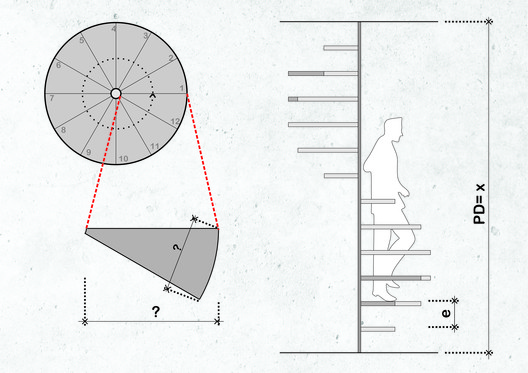# How to Calculate Spiral Staircase Dimensions and Designs

How to Calculate Spiral Staircase Dimensions and Designs

Spiral staircases save valuable square meters because they occupy a much smaller area than a conventional staircase. With daring shapes and diverse configurations, they can also be iconic objects in projects. However, the design of these staircases requires careful attention so that you can prevent an uncomfortable or dangerous outcome. Although BIM software simplifies this process, it's always important to understand the restrictions and the underlying concepts.

Spiral staircases can adopt different structural configurations. The most common ones have a circular format with a central mast from which the steps are fixed.

For this design, three main factors must be taken into account:

• How high must the staircase rise vertically? (Distance between floors)
• What is its angle of rotation?
• What is its diameter?

It's important to be clear about where the staircase will begin and where it will end at the upper level, according to the flows that have been resolved in the plan. You'll also need to find the angle of rotation, which is determined by the stair's diameter. There are a few free online calculators that help simplify this process. To measure the number of steps, it's essential to know the diameter and the length of the staircase. To calculate the total length, use the following formula:

### length of helical staircase = (π (Pi) x radius x angle of rotation) / 180)

However, considering that the steps have irregular shapes as they approach the central mast, it's important to define the point at which you will apply the calculation of the steps of the staircase. It's considered that this "useful line," where the user walks, is located 2/3 from the center.

Having determined this, you can adopt the same method as a conventional staircase, as we indicated in this post. The only difference is that, since there is a greater difficulty in adapting to the Blondel formula, a slightly higher tolerance should be used.

For example, if there is a distance of 3.00 m between slabs and the space for a staircase of 100 cm radius, according to its layout in space, the staircase will start at the same point where it will end, that is, it will have a rotation angle of 360 °. When inserting the values in the previous formula, its total circumference will be 6.28 m.

Using the useful line, you will see that the calculated length is 4.18 meters where people will step on. If we try 15 steps, we will have 27 cm treads and 20 cm risers. Applying the Blondel law, we end up with a total of 67, which would slightly exceed a conventional staircase. In a spiral staircase, with certain restrictions, that value can be accepted.

Although the minimum radius to be considered in a helical staircase is 70 centimeters, you should always give preference to stairs with at least 80 centimeters radius. It must also take into account a minimum height of 2.15 meters between the steps and the roof, or the upper steps.

Following these recommendations, you can have greater autonomy when proposing a spiral staircase. Don't be afraid, free your creativity and design an interesting and functional staircase!## #Tags

Cite: Souza, Eduardo. "How to Calculate Spiral Staircase Dimensions and Designs" 17 Jun 2018. ArchDaily. Accessed . <https://www.archdaily.com/896537/how-to-calculate-spiral-staircase-dimensions-and-designs> ISSN 0719-8884